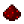# bit.tobitsFunction bit.tobits Converts a number to an array (numerically-indexed table) containing the corresponding binary bit values (the inverse of bit.tonumb) Syntax bit.tobits(number n) Returns table the bits making up the value n, with entries up to the most-significant 1 bit in n Part of ComputerCraft API bitExample Convert the number 18 into its binary representation (10010) Code ```for k, v in pairs(bit.tobits(18)) do print(k, ", ", v) end ``` Output 1, 02, 13, 04, 05, 1